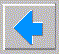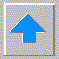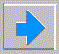# Lecture 4 - Complexity, Godel's theorem, the Halting problem

• Complexity
• Bandersnatch problem (Garey and Johnson)
• problems can be :
• easy (polynomial)
• NP-hard
• truly hard (provably non-polynomial, exponential)
• impossible
• Decision problems (problems with yes/no answers) can be :
• P (problems with poly-time Turing solution)
• NP (can test solution in poly time, so can guess and test)
• NP-complete (hardest problems in NP)
• truly hard (must try all combinations)
• Reduction of problems (if can solve A can certainly solve B)
• Does P=NP? (drawing of world of problems) unknown!
• Examples of NP-complete problems: TSP, SAT, knapsack
• All of the above is worst case analysis, average case may be easy!
• Godel's theorem and the Halting problem
• Russels’s paradox: barber paradox, Epimedes “I am lying”
• Goldbach conjecture (every even>4 = sum of two primes) vs. difference of two primes
• Examples: always, never, sometimes, sometimes2, looper, who_knows Pascal code
• use of string representation (Godel - numeric coding)
• proof that halts function does not exist Pascal code
• Godel’s theorem (every sufficiently strong axiom system has non-provable true theora)
• Eliminating Russel’s paradox from axiomatic set theory
• Principia Mathematicia - all math based on set theory
• Do we know any Godelean problems (FLT, Goldbach, P=NP)?